• 累计撰写 135 篇文章
• 累计创建 25 个标签
• 累计收到 32 条评论

### 目 录CONTENT# 数值的整数次方2021-11-16 / 0 评论 / 0 点赞 / 17 阅读 / 3,216 字

## 实现思路

  /**
* 计算一个数的次方
* @param base 底数
* @param exponent 指数
*/
public power(base: number, exponent: number): number {
let result = 1;
for (let i = 1; i <= exponent; i++) {
result *= base;
}
return result;
}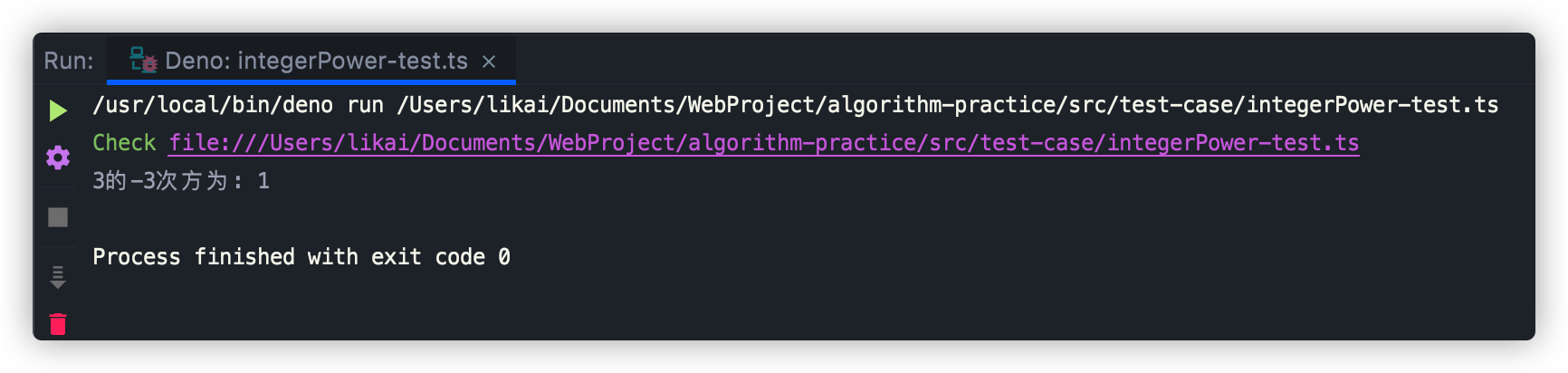### 全面考虑的解法

• 当指数为负数的时候，需要对指数求绝对值，算出次方的结果之后再取倒数
• 当指数为0时，我们就要考虑两种情况：
• 当底数为0且指数为负数时，就会出现对0求倒数，会导致程序运行出错，需要进行容错处理，将错误信息告知调用者
• 当底数为0且指数为0时，这在数学上是没有意义的，此处我们将结果返回0或1都可以

  /**
* 计算一个数的次方
* @param base 底数
* @param exponent 指数
*/
public power(base: number, exponent: number): number | string {
// 求出指数对绝对值
const absExponent = Math.abs(exponent);
// 程序会出错
if (base === 0 && exponent < 0) {
return "参数错误: 0的负次方不能进行计算";
}

// 当底数和指数都为0时，在数学上是没意义的
if (base === 0 && exponent === 0) {
return 0;
}

let result = 1;
for (let i = 1; i <= absExponent; i++) {
result *= base;
}

// 指数小于0, 对计算出的结果求倒数
if (exponent < 0) {
result = 1 / result;
}
return result;
}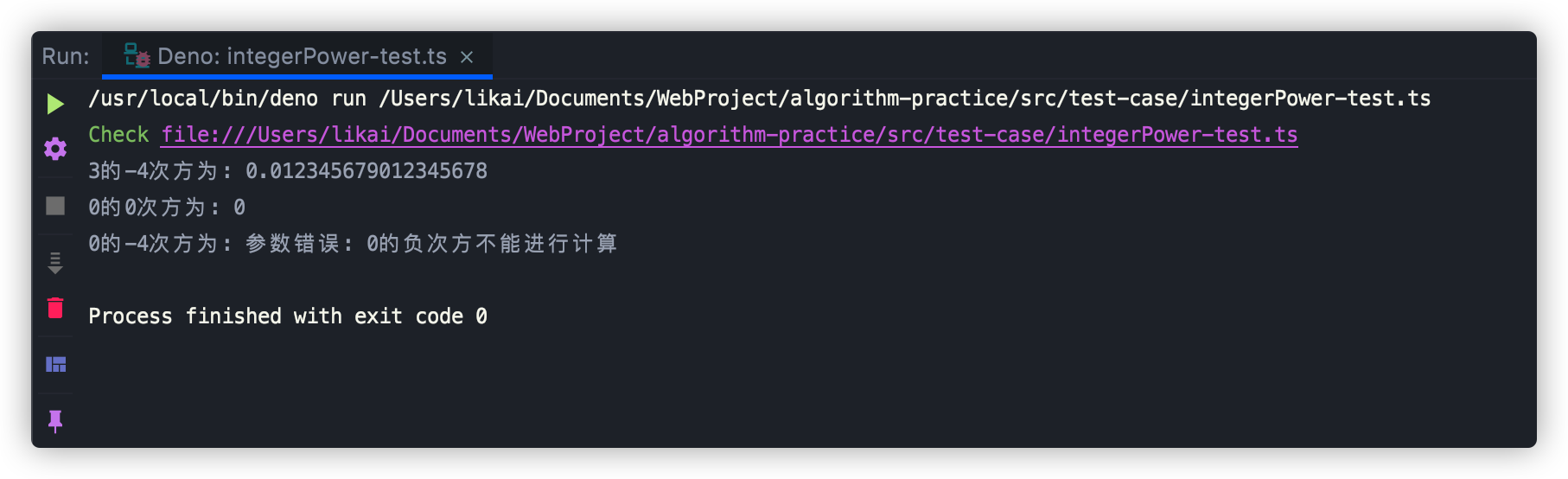### 最优解

  /**
* 求底数的指数次方
* @param base
* @param exponent
*/
private static powerWithExponent(base: number, exponent: number) {
let result = 1;
for (let i = 1; i <= exponent; i++) {
result *= base;
}
return result;
}


• 先求平方
• 在平方的基础上求4次方
• 在4次方的基础上求8次方
• 在8次方的基础上求16次方
• 在16次方的基础上求32次方

• 当n为偶数时，可以拆分为n/2 * n/2
• 当n为奇数时，可以拆分为(n-1)/2 * (n-1)/2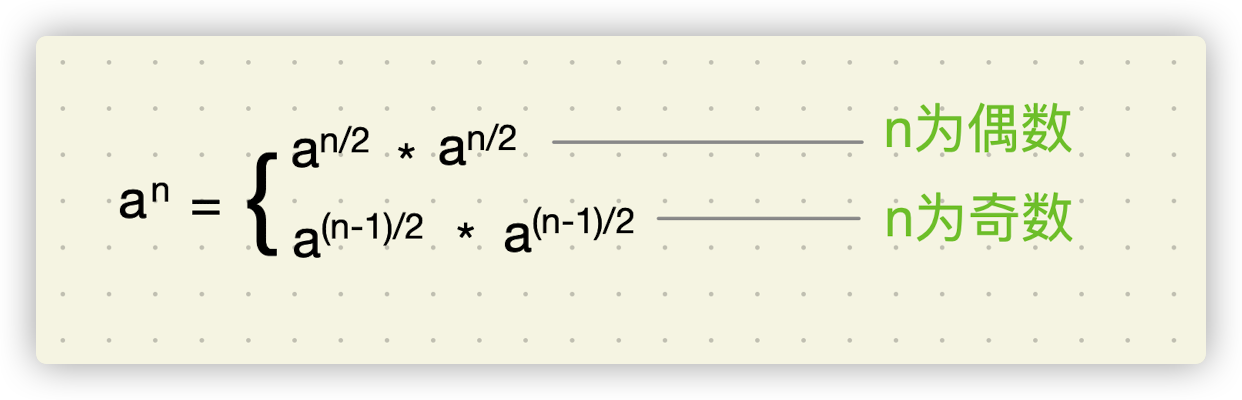#### 实现代码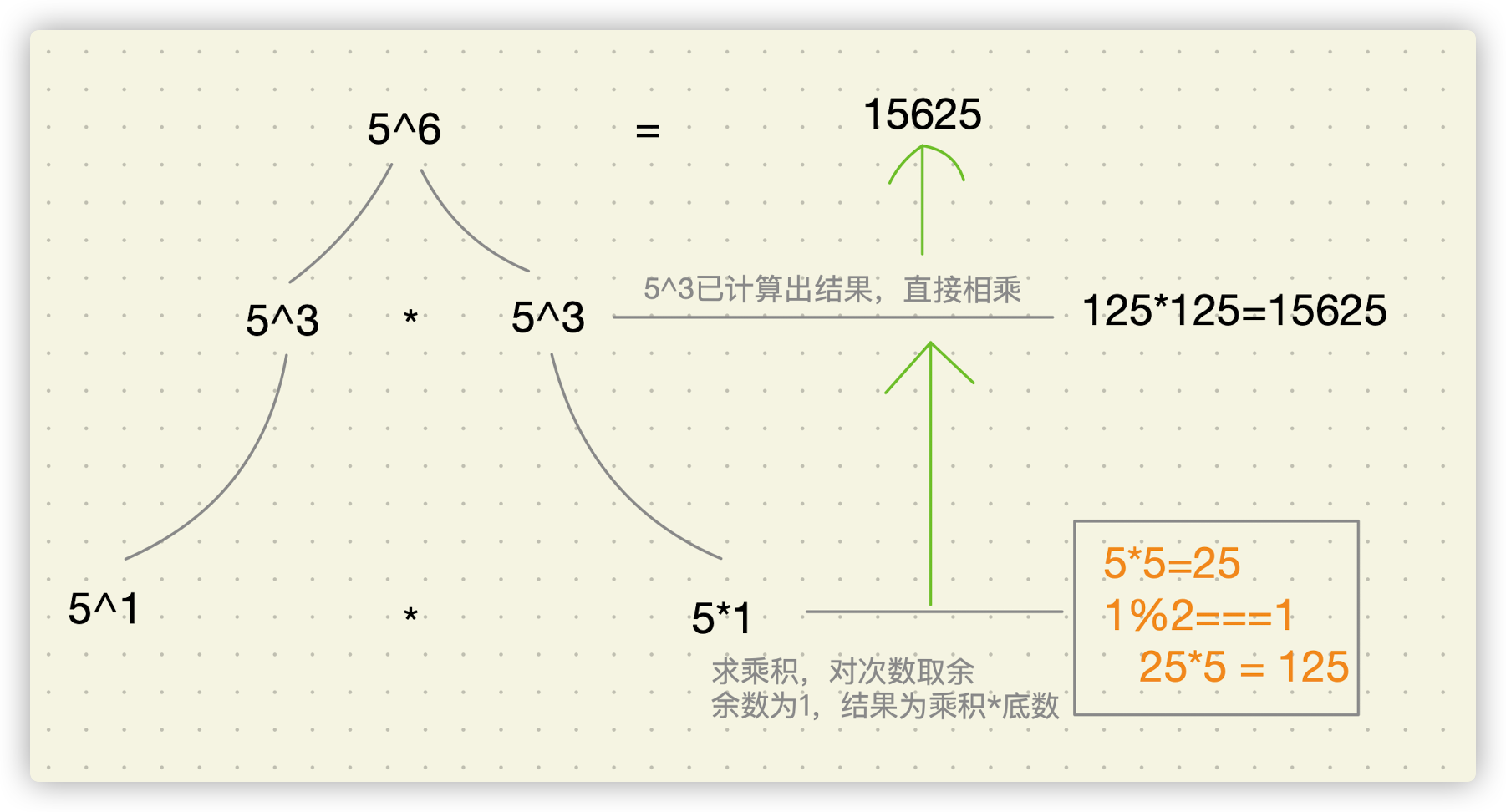export default class IntegerPower {
/**
* 计算一个数的次方
* @param base 底数
* @param exponent 指数
*/
public power(base: number, exponent: number): number | string {
// 求出指数对绝对值
const absExponent = Math.abs(exponent);
// 程序会出错
if (base === 0 && exponent < 0) {
return "参数错误: 0的负次方不能进行计算";
}

// 当底数和指数都为0时，在数学上是没意义的
if (base === 0 && exponent === 0) {
return 0;
}

// 进行次方运算
let result = this.powerWithExponent(base, absExponent);

// 指数小于0, 对计算出的结果求倒数
if (exponent < 0) {
result = 1 / result;
}
return result;
}

/**
* 求底数的指数次方
* @param base 底数
* @param exponent 指数
*/
private powerWithExponent(base: number, exponent: number): number {
// 递归终止条件
if (exponent === 0) {
// 非0数的0次方值为1
return 1;
}
if (exponent === 1) {
// 任意数的0次方为其本身
return base;
}

// 递归对指数/2，计算结果
// 用右移运算符代替除以2运算
let result =
this.powerWithExponent(base, exponent >> 1) *
this.powerWithExponent(base, exponent >> 1);

// 指数为奇数时，result就为result乘以底数
if (exponent % 2 === 1) {
result *= base;
}
return result;
}
}



#### 编写测试用例

import IntegerPower from "../IntegerPower.ts";

const powerHandler = new IntegerPower();
const result1 = powerHandler.power(5, 6);
const result2 = powerHandler.power(3, -4);
const result3 = powerHandler.power(0, 0);
const result4 = powerHandler.power(0, 3);
const result5 = powerHandler.power(0, -3);
console.log(result1);
console.log(result2);
console.log(result3);
console.log(result4);
console.log(result5);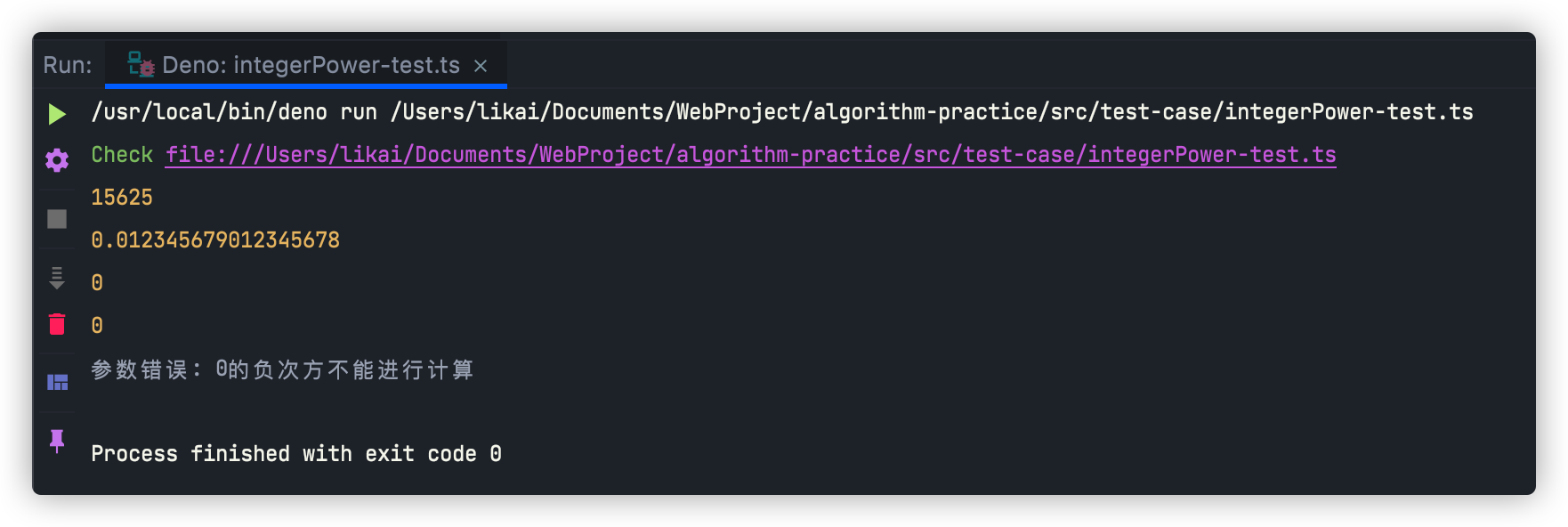## 写在最后

• 文中如有错误，欢迎在评论区指正，如果这篇文章帮到了你，欢迎点赞和关注😊
• 本文首发于神奇的程序员公众号，未经许可禁止转载💌
0